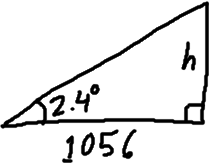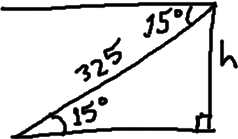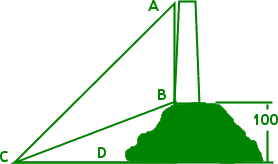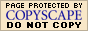Angles of Elevation / Inclination and
Angles of Depression / DeclinationAngles of elevation or inclination are angles above the horizontal, like looking up from ground level toward the top of a flagpole. Angles of depression or declination are angles below the horizontal, like looking down from your window to the base of the building in the next lot. Whenever you have one of these angles, you should immediately start picturing how a right triangle will fit into the description.

• Driving along a straight flat stretch of Arizona highway, you spot a particularly tall saguaro ("suh-WARH-oh") cactus right next to a mile marker. Watching your odometer, you pull over exactly two-tenths of a mile down the road. Retrieving your son's theodolite from the trunk, you measure the angle of elevation from your position to the top of the saguaro as 2.4°. Accurate to the nearest whole number, how tall is the cactus?
• Two-tenths of a mile is 0.2×5280 feet = 1056 feet, so this is my horizontal distance. I need to find the height h of the cactus. So I draw a right triangle and label everything I know:

 The scale is not important; I'm not bothering to get the angle "right". I'm using the drawing as a way to keep track of information; the particular size is irrelevant. What is relevant is that I have "opposite" and "adjacent" and an angle measure. This means I can create and solve an equation: h/1056 = tan(2.4°) h = 1056×tan(2.4°) = 44.25951345...To the nearest foot, the saguaro is 44 feet tall.

• You were flying a kite on a bluff, but you managed somehow to dump your kite into the lake below. You know that you've given out 325 feet of string. A surveyor tells you that the angle of declination from your position to the kite is 15°. How high is the bluff where you and the surveyor are standing?

 First, I draw my triangle: The horizontal line across the top is the line from which the angle of depression is measured. But by nature of parallel lines, the same angle is in the bottom triangle. I can "see" the trig ratios more easily in the bottom triangle, and the height is a bit more obvious. So I'll use this part of the drawing.I have "opposite", hypotenuse, and an angle, so I'll use the sine ratio to find the height. h/325 = sin(15°) Copyright © Elizabeth Stapel 2010-2011 All Rights Reserved h = 325×sin(15°) = 84.11618966... The bluff is about 84 feet above the lake.
 A lighthouse stands on a hill 100 m above sea level. If ∠ACD measures 60° and ∠BCD is 30°, find the height of the lighthouse.I'm going to have to work this exercise in steps. I can't find the height of the tower, AB, until I have the length of the base CD. (Think of D as being moved to the right, to meet the continuation of AB, forming a right triangle.) For this computation, I'll use the height of the hill.

100/|CD| = tan(30°)
100/tan(30°) = |CD| = 173.2050808...

To minimize round-off error, I'll use all the digits from my calculator in my computations, and try to "carry" the computations in my calculator the whole way..

Now that I have the length of the base, I can find the total height, using the angle that measures the the elevation from sea level to the top of the tower.

h/173.2050808 = tan(60°)
h = 173.2050808×tan(60°) = 300

Excellent! By keeping all the digits and carrying the computations in my calculator, I got an exact answer. No rounding! But I do need to subtract, because "300" is the height from the water to the top of the tower. The first hundred meters of this total height is hill, so:

The tower is 200 meters tall.